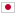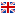Contest Duration: - (local time) (300 minutes)
H - Separate String/Time Limit: 2 sec / Memory Limit: 512 MB

### Problem Statement

You are given a string $t$ and a set $S$ of $N$ different strings. You need to separate $t$ such that each part is included in $S$.

For example, the following 4 separation methods satisfy the condition when $t = abab$ and $S = \{a, ab, b\}$.

• $a, b, a, b$
• $a, b, ab$
• $ab, a, b$
• $ab, ab$

Your task is to count the number of ways to separate $t$. Because the result can be large, you should output the remainder divided by $1{,}000{,}000{,}007$.

### Input

The input consists of a single test case formatted as follows.

$N$ $s_1$ $\vdots$ $s_N$ $t$

The first line consists of an integer $N \ (1 \le N \le 100{,}000)$ which is the number of the elements of $S$. The following $N$ lines consist of $N$ distinct strings separated by line breaks. The $i$-th string $s_i$ represents the $i$-th element of $S$. $s_i$ consists of lowercase letters and the length is between $1$ and $100{,}000$, inclusive. The summation of length of $s_{i} \ (1 \le i \le N)$ is at most $200{,}000$. The next line consists of a string $t$ which consists of lowercase letters and represents the string to be separated and the length is between $1$ and $100{,}000$, inclusive.

### Output

Calculate the number of ways to separate $t$ and print the remainder divided by $1{,}000{,}000{,}007$.

### Sample Input 1

3
a
b
ab
abab


### Output for Sample Input 1

4


### Sample Input 2

3
a
b
c
xyz


### Output for Sample Input 2

0


### Sample Input 3

7
abc
ab
bc
a
b
c
aa
aaabcbccababbc


### Output for Sample Input 3

160


### Sample Input 4

10
a
aa
aaa
aaaa
aaaaa
aaaaaa
aaaaaaa
aaaaaaaa
aaaaaaaaa
aaaaaaaaaa
aaaaaaaaaaaaaaaaaaaaaaaaaaaaaaaaaaaaaaaa


### Output for Sample Input 4

461695029